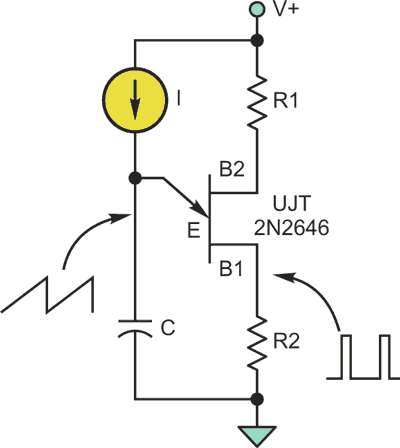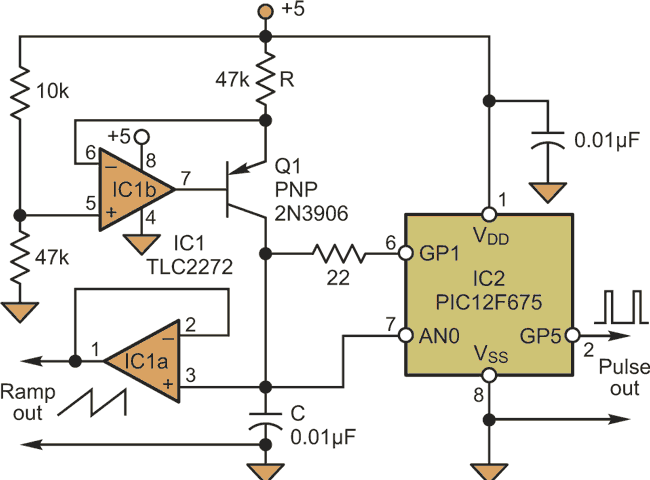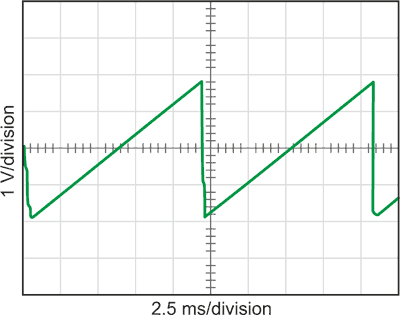# Ramp Generator Uses Microcontroller Emulation Of Unijunction Transistor

Dev Gualtieri

Electronic Design

By using a standard, low-cost PIC microcontroller with a short block of code that emulates a simple ramp-signal generator based on the venerable unijunction transistor (UJT), you can achieve smooth output and infinite resolution without the challenges of finding, using, and controlling a ramp-signal generator.

Unijunction transistors (UJTs) were common circuit elements several decades ago. A simple ramp generator could be built from a single UJT and a few other components (Fig. 1).Figure 1. In a simple UJT-based ramp generator, the current source linearly charges the capacitor to create a voltage ramp. A synchronizing output pulse is also available.

The operating principle is simple. The base-emitter junction is initially in a high-impedance state, and the current source linearly charges the capacitor until a breakdown voltage is reached. At that point, the capacitor discharges through the UJT base until a lower threshold voltage is reached. Then, the base-emitter connection returns to a high-impedance state, and the capacitor can recharge.

This approach still has a few advantages over digital circuitry, as there are tradeoffs when using a digital-to-analog converter (DAC) to create a ramp. Inexpensive microcontrollers include high-resolution analog-to-digital converters (ADCs), but high-resolution DACs are expensive peripheral components. While such DACs have limited resolution, an analog ramp like one produced by a UJT has infinite resolution.

It’s possible to emulate the action of a UJT using just three I/O pins of a microcontroller and a few other components. Most microcontrollers allow their I/O pins to be dynamically reconfigured to function as either a high-impedance input or as an output. This feature is the basis for a simple UJT-emulated ramp generator.

In the microcontroller emulation, the current source is implemented by the rail-to-rail operational amplifier IC1b of a dual device and Q1, while IC1a buffers the voltage on capacitor C and provides the ramp output (Fig. 2). This capacitor is connected to both analog/digital converter pin AN0 of the microcontroller (IC2) and to its I/O pin GP1, which is initially configured as an input. A 22-Ω current-limiting resistor allows high-value capacitors to be used without damage to the microcontroller.Figure 2. The microcontroller-based emulation of a UJT ramp generator switches the function of an I/O pin from a high-impedance input state to an output state to implement the ramp-generation function.

The pseudocode listing for the microcontroller continually monitors the capacitor voltage (see the code).

Pseudo-code For The Microcontroller Emulation Of A UJT Ramp Generator

```
main()
{
initialize_microcontroller();
discharge_pin = high-Z_digital_input;
pulse_pin = 0; //optional pulse output
while(l) //Infinite loop
{
v = analog_voltage(analog_pin);
if (v>v_upper)
{
pulse_pin = 1;
discharge_pin = low-Z_digitaI_output;
while (v>v_lower)
{
//loop until capacitor is discharged
v = analog_voltage(analog_pin);
}
pulse_pin = 0;
discharge_pin = high-Z_digital_input;
}
}
}
```Figure 3. The ramp output repetition rate is about 85 Hz using the values shown. It can be changed via resistor R, which sets the charging current, and capacitor C.

When it reaches a threshold value, it switches the I/O pin from its high-impedance input state to an output state at ground potential. When the capacitor voltage goes below another threshold value, the I/O pin is switched back to a high-impedance state, and the cycle repeats (Fig. 3). The circuit also provides a pulse synchronized with the ramp cycles, which can be used for timing and triggering.

electronicdesign.com